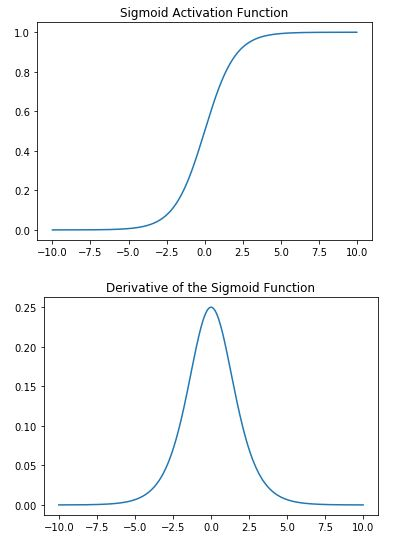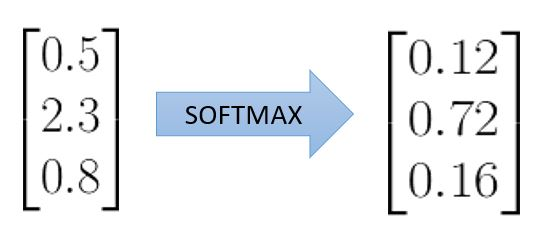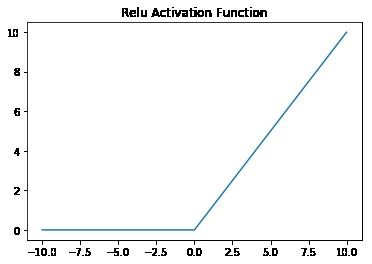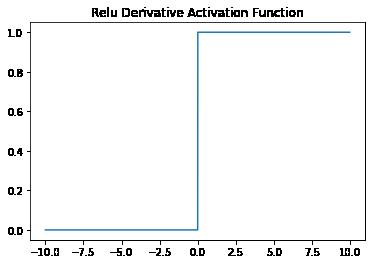## Top 3 Neural Network Activation Functions

Activation functions are an important component of Neural Networks, providing them the ability to learn. When Neural Networks utilize non-linear activation functions, they learn to model complex non-linear relationships making them able to perform tasks we continue to be amazed at in Natural Language Processing, Business Analytics or powering self-driving cars.

In this article we will go over three of the most commonly used activation functions in neural networks but first a quick overview of activation functions.

### Quick Overview of Activation Functions

At a high level, the forward propagation step at each neuron calculates the following weighted sum of the inputs plus the bias:

It then applies an activation function A to generate an output at each neuron:

The activation function performs a nonlinear transformation of Y into a range that will determine if the neuron will fire or not based on some threshold. Stack many of these neurons together and you end up with a neural network.

The nonlinear transformation is an important property of activation functions. If your activation functions where linear, it wouldn’t matter how many layers where in your neural network, the end result would still be a linear function. No sense in using neural networks at all for this.

There are many activation functions each with its pros and cons. The following are the top 3 most commonly used today.

### Sigmoid

The sigmoid activation function is defined as follows:

At first commonly used in hidden and outer layers, it is now mostly used in the outer layers. It’s range between 0 and 1 makes it ideal for binary classification problems.

The usage of the sigmoid function has decreased in hidden layers due to speed. This function experiences what is called the vanishing gradient problem. Take a look at the derivate chart further below. At the negative and positive extremes the derivative is close to 0. This poses a problem to deep neural networks as the model stops learning or learns at a very small rate which exponentially increases the training time.

Backpropagation is also not optimized when using the sigmoid activation function. In your preprocessing steps you usually normalize the values to a mean of 0 in order to increase the efficiency of gradient decent. The sigmoid function centered at 0.5 doesn’t help in this. Our next function takes aim to fix this.

Due to the above, the sigmoid function is now mostly used in the outer layers for binary classification tasks.The vectorized python implementation of the sigmoid function is as follows:

def sigmoid(x):
return 1 / (1 + np.exp(-x))

def sigmoid_derivative(x):
return sigmoid(x) * (1-sigmoid(x))

### Softmax

Next up in our top 3 activation functions list is the Softmax function. This function is commonly used in the output layer of neural networks when dealing with a multi class classification problem.

Like the sigmoid function, the Softmax transforms its inputs into a range between 0 and 1. It divides e raised to each item by the exponential sum of all the classes to normalize the output. The result is a categorical probability distribution.

The class most likely to be true is that which has the highest probability. Easy to see why this is the function of choice for multi-class classification in the output layers, to transform the network’s outputs into a categorical probability distribution.

The formula of the Softmax is as follows:

Where z is a vector, K is the vector dimensions and j is the ith  element of the vector.To implement the softmax function in python you would do the following vectorized implementation:

import numpy as np
def softmax(z):
return np.exp(z)/np.sum(np.exp(z))

If we pass along the vector of the above image to this function, you will get the same result:

z=[0.5,2.3,0.8]
softmax(z)
array([ 0.11905462,  0.72023846,  0.16070692])

### RELU

RELU or Rectified Linear Unit are commonly used today in the hidden layers of neural networks.

They are one of the reasons machine learning practitioners have been able to train deeper neural nets. RELU’s don’t exhibit the vanishing gradient problem described previously, allowing researches to train large neural networks in a much faster time.

The formula for the Relu is as follows:

The derivative of the RELU is always 1 or 0, excluding the derivative at 0 which is undefined but commonly replaced with 1, meaning the derivative doesn’t become extremely small at outer ranges as is the case for the sigmoid function or other activation functions such as the tanh.Not everything is perfect about RELU’s, they can exhibit a different issue called the Dying Relu problem, particularly when the learning rate is too high. In short, a large gradient can cause a weight update that causes the RELU to not activate anymore at any other data point. Therefore, the neuron exhibiting this never fires again and is said to be dead.

Even though there is the possibility of the Dying Relu problem, the rule of thumb is to use RELU’s in your hidden layers unless you have a good reason not to.

To implement the RELU in python use the below code:

def relu(x):
return x * (x > 0)

def relu_derivative(x):
return np.where(x>0,1,0)

### Conclusion

We have now gone over the 3 most commonly used activation functions in neural networks and you can use the following rules of thumb when implementing your algorithms, particularly if you are just starting out and don’t know where to start.

• For binary classifications, use the sigmoid function in the outer layer.
• For multi-label classifications, use the sigmoid function in the outer layer.
• For multi-class classifications, use the softmax function in the outer layer.
• For all hidden layers, use the RELU.